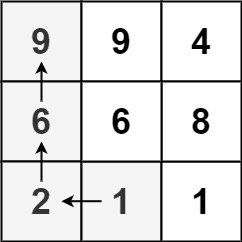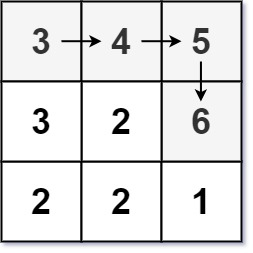329. Longest Increasing Path in a Matrix
Hard
8.1K
119

Given an `m x n` integers `matrix`, return the length of the longest increasing path in `matrix`.

From each cell, you can either move in four directions: left, right, up, or down. You may not move diagonally or move outside the boundary (i.e., wrap-around is not allowed).

Example 1:```Input: matrix = [[9,9,4],[6,6,8],[2,1,1]]
Output: 4
Explanation: The longest increasing path is `[1, 2, 6, 9]`.
```

Example 2:```Input: matrix = [[3,4,5],[3,2,6],[2,2,1]]
Output: 4
Explanation: The longest increasing path is `[3, 4, 5, 6]`. Moving diagonally is not allowed.
```

Example 3:

```Input: matrix = []
Output: 1
```

Constraints:

• `m == matrix.length`
• `n == matrix[i].length`
• `1 <= m, n <= 200`
• `0 <= matrix[i][j] <= 231 - 1`
Accepted
447.9K
Submissions
852.3K
Acceptance Rate
52.6%

Seen this question in a real interview before?
1/4
Yes
No

Discussion (0)

Related Topics